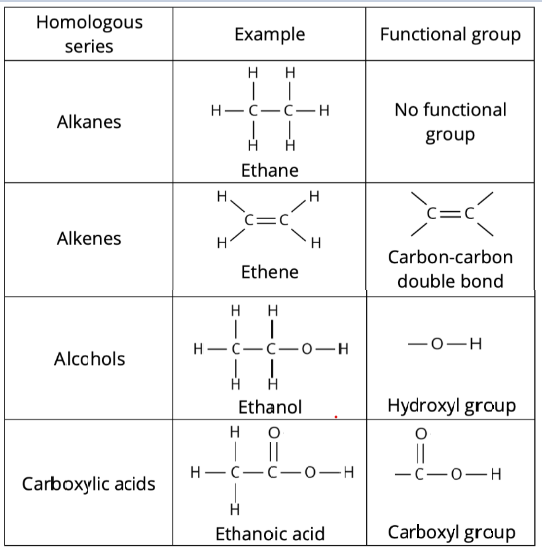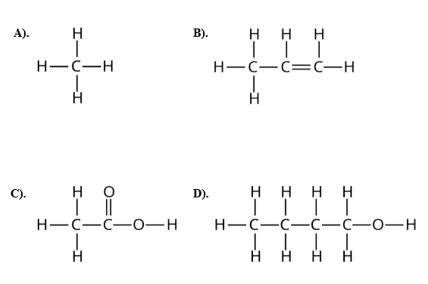Study S4 Chemistry Introduction to Organic Chemistry - Geniebook# Introduction To Organic Chemistry

In the following article, we will cover the topic on Introduction to Organic Chemistry. These are the following learning outcomes:

• Define a homologous series.
• Describe a functional group.
• Naming organic compounds.

## Organic Chemistry

Organic chemistry is the chemistry of carbon-containing compounds.

Organic compounds are compounds that contain the element carbon. Most organic compounds also contain hydrogen.

Organic compounds that contain only hydrogen and carbon are called hydrocarbons. E.g. methane (CH4)

Some organic compounds may also contain oxygen, nitrogen and halogens.

However, not all compounds that contain carbon are organic compounds. Compounds such as:

• carbon dioxide and carbon monoxide (CO2 and CO)
• metal carbonates,

are not organic compounds, even though they contain carbon atom(s).

In the field of chemistry, organic compounds are referred to as compounds that have been derived from living things.

## Homologous Series

A homologous series is a family of organic compounds with the same functional group and similar chemical properties.

Examples of homologous series:

• alkanes
• alkenes
• alcohols
• carboxylic acids

### Functional Group

A functional group is an atom or group of atoms that gives a molecule its characteristic properties.

Organic compounds in the same homologous series have similar chemical properties because they have the same functional groups.

The table below shows the functional groups of the four different homologous series.### Characteristics Of Homologous Series

Organic compounds in the same homologous series have the following characteristics:

• They have the same functional group.
• They have similar chemical properties.
• They show a gradual change in their physical properties (e.g. melting and boiling point, density, viscosity, flammability) as we move from one member to the next.

### Naming Organic Compounds

The name of an organic compound consists of two parts – a prefix and a suffix.

The prefix tells us the number of carbon atoms in the molecule.

Number Of Carbon Atoms Per Molecule Prefix
one meth-
two eth-
three prop-
four but-

The suffix tells us the homologous series of the compound.

Homologous Series Suffix
alkane -ane
alkene -ene
alcohol -ol
carboxylic acid -oic acid

Note: For alcohol and carboxylic acid, ‘an’ is added in front of ‘ol’ and ‘oic acid’ respectively to make it sound better.

Example 1:

Let us practise naming the following organic compounds.Solution:

1. There is only one carbon atom in the molecule. This molecule belongs to the alkane homologous series, as the molecule does not contain any functional group. There are only C-C and C-H bonds present. The prefix is ‘meth-’ (one carbon atom) and the suffix is ‘-ane’ (belongs to the alkane family). The name of this molecule is thus methane.
2. There are three carbon atoms in the molecule. This molecule belongs to the alkene homologous series, as the molecule contains the carbon-carbon double bond as its functional group. The prefix is ‘prop-’ (three carbon atoms) and the suffix is ‘-ene’ (belongs to the alkene family). The name of this molecule is thus propene.
3. There are two carbon atoms in the molecule. This molecule belongs to the carboxylic acid homologous series, as the molecule contains the carboxyl group (-COOH) as its functional group. The prefix is ‘eth-’ (two carbon atoms) and the suffix is ‘-oic acid’ (belongs to the carboxylic acid). The name of this molecule is thus ethanoic acid.
4. There are four carbon atoms in the molecule. This molecule belongs to the alcohol homologous series, as the molecule contains the hydroxyl group (-OH) as its functional group. The prefix is ‘but-’ (four carbon atoms) and the suffix is ‘-ol’ (belongs to the alcohol family). The name of this molecule is thus butanol

### Game Section

Question1:

Can you name the following organic compound?1. propane
2. butane
3. ethanol
4. butene

Solution:

(B) butane

There are four carbon atoms in the molecule, so the prefix is ‘but-’. This molecule does not contain any functional group. There are only C-C and C-H bonds present. Hence it belongs to the alkane homologous series. The suffix is ‘-ane’. Thus, the name of this compound is butane.

Question 2:

Can you name the following organic compound ?1. methanol
2. butanoic Acid
3. ethene
4. propane

Solution:

(C) ethene

Explanation:

There are two carbon atoms in the molecule, so the prefix is ‘eth-’. This molecule belongs to the alkene homologous series, as the molecule contains the carbon-carbon double bond as its functional group. The suffix is ‘-ene’. Thus, the name of this compound is ethene.

Question 3 :

Can you name the following organic compound?1. ethanol
2. propanol
3. ethanoic acid
4. propanoic acid

Solution:

(D) propanoic acid

Explanation:

There are three carbon atoms in the molecule, so the prefix is ‘prop-’. This molecule belongs to the carboxylic acid homologous series, as the molecule contains the carboxyl group (-COOH) as its functional group. The suffix is ‘-oic acid’. Thus, the name of this compound is propanoic acid.

Question 4:

Can you identify the functional group(s) in this organic compound?Solution:

There are 2 functional groups:

• carbon-carbon double bond (C=C)
• carboxyl group (-COOH)

Example 2:

A student commented that the compounds below belong to the same homologous series. Do you agree? Explain.Solution:

Yes. The two compounds belong to the same homologous series, as they have same functional group, which is the carbon-carbon double bond (C=C).

## Try It Yourself

Example:

Given below are three organic compounds.Two of these compounds belong to the same homologous series. Identify them and explain.

Solution:

The first and third compound belong to the same homologous series of alcohols. They have the same functional group, which is the hydroxyl group (-OH).

Question 1:

Which statements about the homologous series are correct?

1. All members have similar chemical reactions.
2. All members have the same functional group.
3. All members have the same physical properties.

1. A and B only
2. A and C only
3. B and C only
4. A, B and C

Solution:

(A) A and B only

Explanation:

Organic compounds in the same homologous series have the following characteristics:

• They have the same functional group.
• They have similar chemical properties.
• There is a gradual change in their physical properties as we go down the series from one member to the next.

Question 2:

Which list shows a homologous series?Solution:

(1)

Explanation:

A homologous series is a family of organic compounds with the same functional group and similar chemical properties. The compounds in list 1 belong to the same alkane homologous series. Their names contain the same suffix, which is ‘-ane’.

Question 3:

The figures below are the structures of four organic compounds.Identify the compounds that belong to the same homologous series.

1. A and B
2. B and C
3. A, B and D
4. A, B, C and D

Solution:

(A) A and B

Explanation:

Both compounds A and B contain the same functional group, which is the hydroxyl group (-OH). Hence, they belong to the alcohol homologous series. Compound A is ethanol, while compound B is methanol.

Question 4a:

The figures below show four organic compounds.What is the correct sequence of the homologous series (from A to D) to which these four organic compounds belong?

1. alkane, alkene, alcohol, carboxylic acid
2. alkene, alcohol, carboxylic acid, alkane
3. alcohol, alkane, alkene, carboxylic acid
4. alkene, alcohol, alkane, carboxylic acid

Solution:

(D) alkene, alcohol, alkane, carboxylic acid

Explanation:

Observe the functional group present in each of the four organic compounds to identify the homologous series they each belong to.
Compound A contains the carbon-carbon double bond. Hence, it belongs to the alkene homologous series.
Compound B contains the hydroxyl group (-OH). Hence, it belongs to the alcohol homologous series.
Compound C does not contain any functional group. There are only C-C and C-H bonds present. Hence, it belongs to the alkane homologous series.
Compound D contains the carboxyl group (-COOH). Hence, it belongs to the carboxylic acid homologous series.

Question 4b:

The figures below show four organic compounds.What is the name of organic compound B?

1. ethanoic acid
2. methanol
3. methane
4. propene

Solution:

(B) methanol

Explanation:

Compound B contains the hydroxyl group (-OH). Hence, it belongs to the alcohol homologous series. Its molecule contains only one carbon atom. The prefix is ‘meth-’ (one carbon atom) and the suffix is ‘-ol’ (belongs to the alcohol series).

Continue Learning
Metals Introduction to Organic Chemistry
Alcohols Speed of Reaction
Electrolysis Energy Changes
Ammonia Alkanes
Alkenes Carboxylic AcidsPrimaryPrimary 1Primary 2Primary 3Primary 4Primary 5Primary 6SecondarySecondary 1Secondary 2Secondary 3Secondary 4PhysicsChemistry
Carboxylic Acids
Metals
Introduction to Organic Chemistry
Alcohols
Speed Of Reaction
Electrolysis
Energy Changes
Ammonia
Alkanes
AlkenesMaths